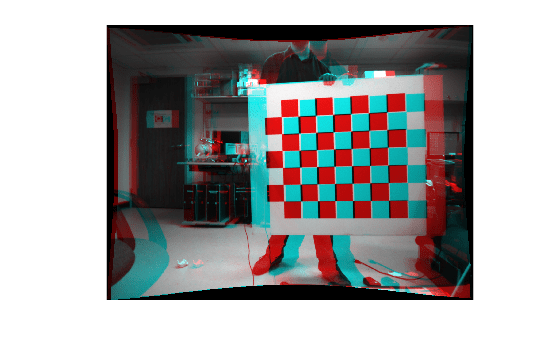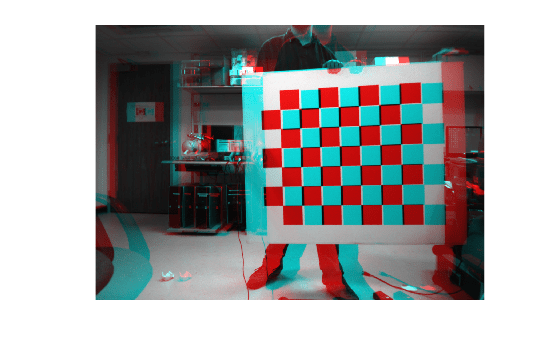# rectifyStereoImages

Rectify a pair of stereo images

## Syntax

``````[J1,J2,reprojectionMatrix] = rectifyStereoImages(I1,I2,stereoParams)``````
``````[___,camMatrix1,camMatrix2,R1,R2] = rectifyStereoImages(I1,I2,stereoParams)``````
``````[J1,J2] = rectifyStereoImages(I1,I2,tform1,tform2)``````
``````[J1,J2] = rectifyStereoImages(___,interp)``````
``````[J1,J2] = rectifyStereoImages(___,Name=Value)``````

## Description

example

``````[J1,J2,reprojectionMatrix] = rectifyStereoImages(I1,I2,stereoParams)``` undistorts and rectifies versions of `I1` and `I2` input images using the stereo parameters of a stereo camera system stored in the `stereoParams` object. Use the `reconstructScene` function with the `reprojectionMatrix` to reproject a 2-D point in a disparity map to a 3-D point in the rectified camera coordinate system of camera 1.Stereo image rectification projects images onto a common image plane in such a way that the corresponding points have the same row coordinates. This image projection makes the image appear as though the two cameras are parallel. Use the `disparityBM` or `disparitySGM` functions to compute a disparity map from the rectified images for 3-D scene reconstruction.```
``````[___,camMatrix1,camMatrix2,R1,R2] = rectifyStereoImages(I1,I2,stereoParams)``` returns the 3-by-4 camera projection matrices `camMatrix1` and `camMatrix2` for the rectified cameras, and the corresponding rectification rotation matrices, `R1` and `R2`.```
``````[J1,J2] = rectifyStereoImages(I1,I2,tform1,tform2)``` returns rectified versions of `I1` and `I2` input images by applying projective transformations `tform1` and `tform2`. The projective transformations are returned by the `estimateUncalibratedRectification` function.```

example

``````[J1,J2] = rectifyStereoImages(___,interp)``` additionally specifies the interpolation method to use for rectified images. You can specify the method as `'nearest'`, `'linear'`, or `'cubic'`.```

example

``````[J1,J2] = rectifyStereoImages(___,Name=Value)``` specifies options using one or more name-value arguments in addition to any combination of arguments from previous syntaxes. For example, `OutputView='valid'` sets the `OutputView` argument to `'valid'`.```

## Examples

collapse all

Specify images containing a checkerboard for calibration.

```imageDir = fullfile(toolboxdir('vision'),'visiondata', ... 'calibration','stereo'); leftImages = imageDatastore(fullfile(imageDir,'left')); rightImages = imageDatastore(fullfile(imageDir,'right'));```

Detect the checkerboards.

```[imagePoints,boardSize] = detectCheckerboardPoints(... leftImages.Files,rightImages.Files);```

Specify world coordinates of checkerboard keypoints.

```squareSizeInMillimeters = 108; worldPoints = generateCheckerboardPoints(boardSize,squareSizeInMillimeters);```

```I1 = readimage(leftImages,1); I2 = readimage(rightImages,1); imageSize = [size(I1,1),size(I1,2)];```

Calibrate the stereo camera system.

`stereoParams = estimateCameraParameters(imagePoints,worldPoints,ImageSize=imageSize);`

Rectify the images using 'full' output view.

`[J1_full,J2_full] = rectifyStereoImages(I1,I2,stereoParams,OutputView='full');`

Display the result for 'full' output view.

```figure; imshow(stereoAnaglyph(J1_full,J2_full));```Rectify the images using 'valid' output view. This is most suitable for computing disparity.

`[J1_valid,J2_valid] = rectifyStereoImages(I1,I2,stereoParams,OutputView='valid');`

Display the result for 'valid' output view.

```figure; imshow(stereoAnaglyph(J1_valid,J2_valid));```## Input Arguments

collapse all

Input image corresponding to camera 1, specified as an M-by-N-by-3 truecolor image or an M-by-N 2-D grayscale array. Input images `I1` and `I2` must also be real, finite, and nonsparse. The input images must be the same class.

Data Types: `uint8` | `uint16` | `int16` | `single` | `double` | `logical`

Input image corresponding to camera 2, specified as an M-by-N-by-3 truecolor image or an M-by-N 2-D grayscale array. Input images `I1` and `I2` must be real, finite, and nonsparse. The input images must also be the same class.

Data Types: `uint8` | `uint16` | `int16` | `single` | `double` | `logical`

Stereo camera system parameters, specified as a `stereoParameters` object.

Data Types: `uint8` | `uint16` | `int16` | `single` | `double`

Projective transformations for image 1, specified as a 3-by-3 matrix returned by the `estimateUncalibratedRectification` function or a `projective2d` object.

Data Types: `single` | `double`

Projective transformations for image 2, specified as a 3-by-3 matrix returned by the `estimateUncalibratedRectification` function or a `projective2d` object.

Data Types: `single` | `double`

Interpolation method, specified as either `'linear'`, `'nearest'`, or `'cubic'`.

### Name-Value Arguments

Specify optional pairs of arguments as `Name1=Value1,...,NameN=ValueN`, where `Name` is the argument name and `Value` is the corresponding value. Name-value arguments must appear after other arguments, but the order of the pairs does not matter.

Example: `OutputView='valid'` sets the `OutputView` argument to `'valid'`.

Size of rectified images, specified as the comma-separated pair consisting of '`OutputView`' and either `'full'` or `'valid'`. When you set this parameter to `'full'`, the rectified images include all pixels from the original images. When you set this value to `'valid'`, the output images are cropped to the size of the largest common rectangle containing valid pixels.

When there is no overlap between rectified images, set the `OutputView` to `'full'`.

Output pixel fill values, specified as the comma-separated pair consisting of '`FillValues`' and an array of one or more scalar values. When the corresponding inverse-transformed location in the input image is completely outside the input image boundaries, use the fill values for output pixels. If `I1` and `I2` are 2-D grayscale images, then you must set '`FillValues`' to a scalar. If `I1` and `I2` are truecolor images, then you can set '`FillValues`' to a scalar or a 3-element vector of RGB values.

## Output Arguments

collapse all

Undistorted and rectified version of `I1`, returned as an M-by-N-by-3 truecolor image or as an M-by-N 2-D grayscale image.

Stereo image rectification projects images onto a common image plane in such a way that the corresponding points have the same row coordinates. This image projection makes the image appear as though the two cameras are parallel. Use the `disparityBM` or `disparitySGM` functions to compute a disparity map from the rectified images for 3-D scene reconstruction.

Undistorted and rectified version of `I2`, returned as an M-by-N-by-3 truecolor image or as an M-by-N 2-D grayscale image.

Stereo image rectification projects images onto a common image plane in such a way that the corresponding points have the same row coordinates. This image projection makes the image appear as though the two cameras are parallel. Use the `disparityBM` or `disparitySGM` functions to compute a disparity map from the rectified images for 3-D scene reconstruction.

Reprojection matrix, returned as a 4-by-4 matrix of the form:

`${\left[\begin{array}{cccc}1& 0& 0& -cx\\ 0& 1& 0& -cy\\ 0& 0& 0& f\\ 0& 0& 1/b& 0\end{array}\right]}_{,}$`

where f and [cx,cy] are the focal length and principal point of rectified camera 1, respectively. b is the baseline of the virtual rectified stereo camera.

Use the `reconstructScene` function with the `reprojectionMatrix` to reproject a 2-D point in a disparity map to a 3-D point in the rectified camera coordinate system of camera 1.

Rectified camera one projection matrix, returned as 3-by-4 matrix. Use `camMatrix1` and `camMatrix2` to project 3-D world points in camera one's coordinate system into the image plane of `J1` and `J2`, respectively.

Use `camMatrix1` to project 3-D world points in the rectified camera one coordinate system into the image plane of `J1`.

Data Types: `single` | `double`

Camera two rectified projection matrix, returned as 3-by-4 matrix. Use `camMatrix1` and `camMatrix2` to project 3-D world points in camera one's coordinate system into the image plane of `J1` and `J2`, respectively.

Use `camMatrix2` to project 3-D world points in the rectified camera two coordinate system into the image plane of `J2`.

Data Types: `single` | `double`

Camera one rotation matrix related to camera one rectified projection, returned as a 3-by-3 matrix. The `R1` rotation matrix relate 3-D points from the unrectified camera one coordinate system to points in the rectified camera one coordinate system.

Data Types: `single` | `double`

Camera two rotation matrix related to camera one rectified projection, returned as a 3-by-3 matrix. The `R2` rotation matrix relate 3-D points from the unrectified camera two coordinate system to points in the rectified camera two coordinate system.

Data Types: `single` | `double`

## Tips

• The Computer Vision Toolbox™ rectification algorithm requires that the epipole for each image lie outside of the image. If the epipole lies within the image, you can first transform the images into polar coordinates as described in the rectification method proposed by Marc Pollefeys, Reinhard Koch, and Luc Van Gool .

 G. Bradski and A. Kaehler, Learning OpenCV : Computer Vision with the OpenCV Library. Sebastopol, CA: O'Reilly, 2008.